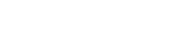# math.tan

`FLOATmath.tanFLOATx`

Available inall subroutines.

Computes the tangent of its argument `x`, measured in radians.

## Parameters

`x` - Floating point value representing an angle in radians.

## Return Value

Upon successful completion, this function returns the tangent of `x`.

If `x` is `math.NAN`, a NaN will be returned.

If `x` is ±0, `x` will be returned.

If `x` is `math.POS_INFINITY` or `math.NEG_INFINITY`, a domain error occurs and a NaN will be returned.

If `x` is subnormal, a range error occurs and `x` will be returned.

If the result would cause an overflow, a range error occurs and `math.tan()` will return `math.POS_HUGE_VAL` or `math.NEG_HUGE_VAL`, with the same sign as the result of the function.

## Errors

• If the `x` argument is `math.POS_INFINITY` or `math.NEG_INFINITY`, then `fastly.error` will be set to `EDOM`.
• If the `x` argument is subnormal or if the result overflows, then `fastly.error` will be set to `ERANGE`.

## Example

``````declare local var.fo FLOAT;
set var.fo = math.tan(math.PI_4);``````

BETA